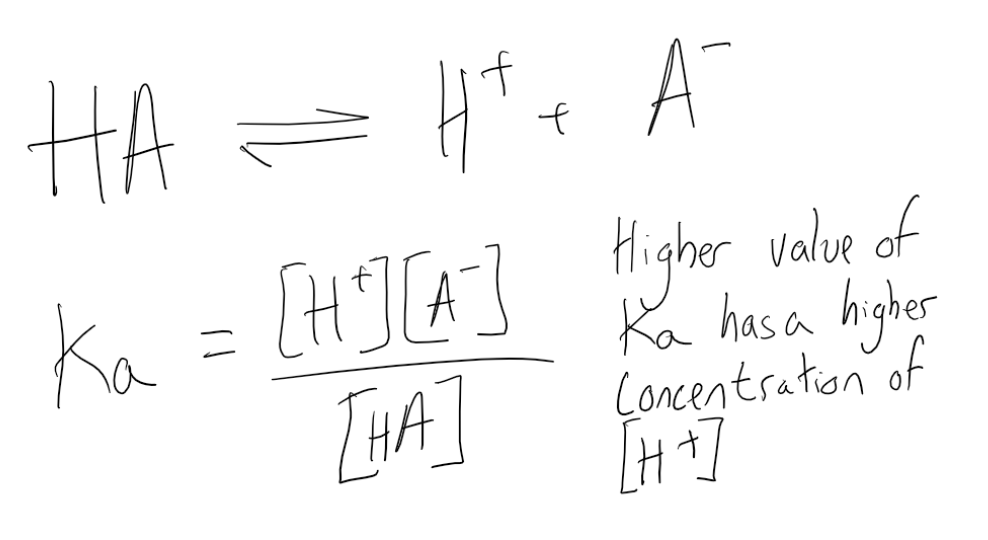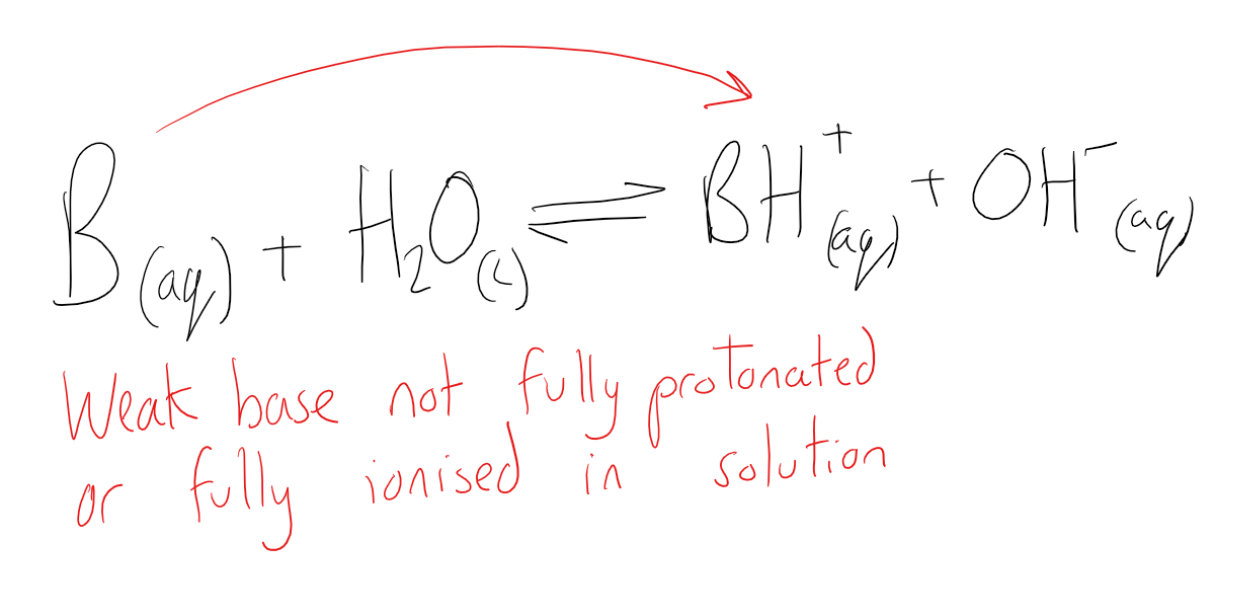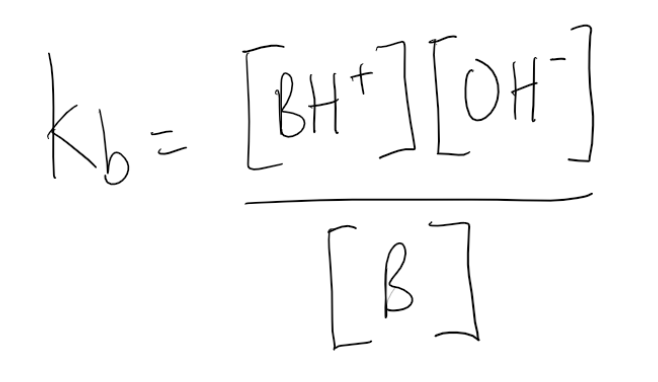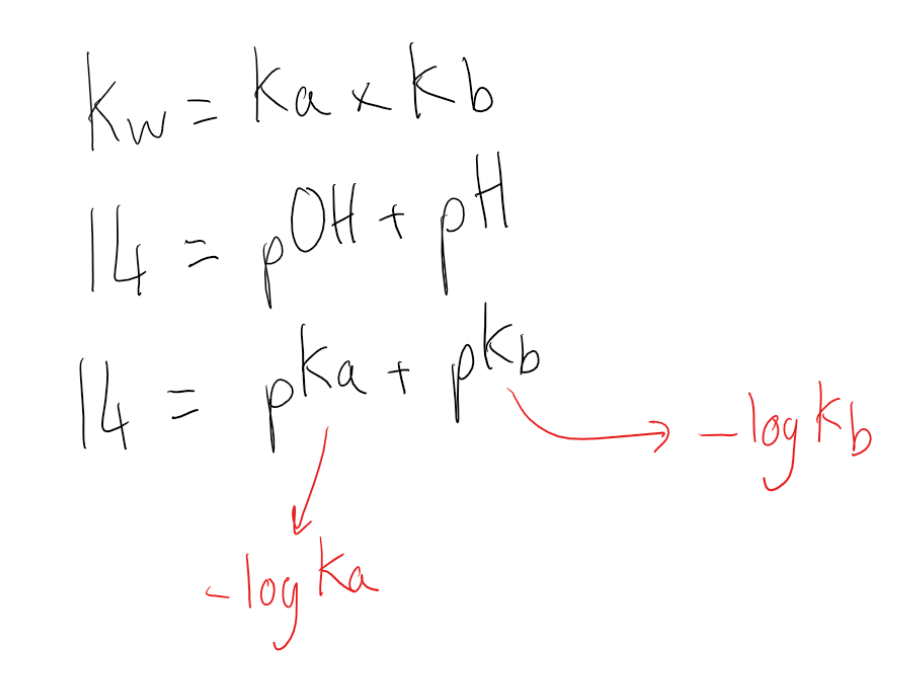Cyber Monday Sale: ALL MCAT Products & Admissions Services

MCAT Content / Acid Base Equilibria / Equilibrium Constants Ka And Kb Pka Pkb

### Equilibrium Constants Ka and Kb: pKa, pKb

Topic: Acid Base Equilibria

The acid dissociation constant (Ka) is a quantitative measure of the strength of an acid in solution while the base dissociation constant (Kb) is a measure of basicity—the base’s general strength.

Ka and pKa
Acids are classified as either strong or weak, based on their ionization in water. A strong acid is an acid which is completely ionized in an aqueous solution. A weak acid is an acid that ionizes only slightly in an aqueous solution.

The ionization for a general weak acid, HA, can be written as follows:Because the acid is weak, an equilibrium expression can be written. An acid ionization constant (Ka) is the equilibrium constant for the ionization of an acid.The acid ionization represents the fraction of the original acid that has been ionized in solution. Therefore, the numerical value of Ka is a reflection of the strength of the acid. Weak acids with relatively higher Ka values are stronger than acids with relatively lower Ka values. Because strong acids are essentially 100% ionized, the concentration of the acid in the denominator is nearly zero and the Ka value approaches infinity. For this reason, Ka values are generally reported for weak acids only.

The logarithmic constant (pKa) is equal to -log10(Ka). The larger the value of pKa, the smaller the extent of dissociation. A weak acid has a pKa value in the approximate range of -2 to 12 in water. Acids with a pKa value of less than about -2 are said to be strong acids.Kb and pKb
As with acids, bases can either be strong or weak, depending on their extent of ionization. A strong base is a base, which ionizes completely in an aqueous solution. A weak base is a base that ionizes only slightly in an aqueous solution.An equilibrium expression can be written for the reactions of weak bases with water. Because the concentration of water is extremely large and virtually constant, the water is not included in the expression. A base ionization constant (Kb) is the equilibrium constant for the ionization of a base.The numerical value of Kb is a reflection of the strength of the base. Weak bases with relatively higher Kb values are stronger than bases with relatively lower Kb values. It is related to the acid dissociation constant, Ka, by the simple relationship pKa + pKb = 14, where pKb and pKa are the negative logarithms of Kb and Ka, respectively. The base dissociation constant can be expressed as follows:

pKb can be calculated by pKb = -log10(Kb). A large Kb value indicates the high level of dissociation of a strong base. A lower pKb value indicates a stronger base.Because these reactions occur in aqueous solutions, water is not included in the equation even though it is part of the reaction. It is considered the solvent in these reactions, so the concentration stays essentially constant.

Practice Questions

MCAT Official Prep (AAMC)

Chemistry Question Pack Passage 13 Question 77

Chemistry Question Pack Passage 16 Question 88

Sample Test C/P Section Passage 6 Question 30

Practice Exam 1 C/P Section Question 13

Practice Exam 3 C/P Section Question 12

Practice Exam 4 C/P Section Passage 1 Question 2

Practice Exam 4 C/P Section Passage 4 Question 18

Key Points

• Ka the acid dissociation constant and Kb the base dissociation constant are measures of the extend of dissociation of a weak acid or base in equilibrium.

• pKa and pKb are the logarithmic scales of Ka and Kb.

• Kb is related to the acid dissociation constant, Ka, by the simple relationship pKa + pKb = 14, where pKb and pKa are the negative logarithms of Kb and Ka, respectively.

• Kb and Ka are also related through the ion constant for water, Kw, by the relationship Kw = Kb x Kb=a

Key Terms

Dissociation: the process by which compounds split into smaller constituent molecules, usually reversibly.

Weak acid: one that dissociates incompletely, donating only some of its hydrogen ions into solution

Weak base: a proton acceptor that does not ionize fully in an aqueous solution

Equilibrium: The state of a reaction in which the rates of the forward and reverse reactions are equal.

pKa: A quantitative measure of the strength of an acid in solution; a weak acid has a pKa value in the approximate range −2 to 12 in water and a strong acid has a pKa value of less than about −2.

Base ionization constant (Kb): The equilibrium constant for the ionization of a base.

Billing Information